• 图像分割，图像轮廓手动分割，可活动轮廓曲线值，matlab代码
• matlab图像分割肿瘤代码 imtool3D 这是一种旨在查看图像切片的3D堆栈的图像查看器。 例如，如果您将一个DICOM系列CT或MRI图像加载到matlab中，则可以使用此工具轻松地可视化图像。 它使您可以滚动切片，调整窗口和...
•matlab
• MATLAB图像分割之阈值分割

千次阅读 2021-12-02 14:20:52
1、全局阈值分割 可以通过图像全局的信息，例如整个图像的灰度直方图。如果在整个图像中只使用一个阈值，则这种方法叫做全局阈值法，整个图象分成两个区域，即目标对象(黑色)和背景对象(白色)。全局阈值将整个图像...

1、全局阈值分割

可以通过图像全局的信息，例如整个图像的灰度直方图。如果在整个图像中只使用一个阈值，则这种方法叫做全局阈值法，整个图象分成两个区域，即目标对象(黑色)和背景对象(白色)。全局阈值将整个图像的灰度阈值设置为常数。

figure,imshow(I);
I2=rgb2gray(I);%对图像灰度化
T=mean2(I2);% 对图像所有元素（每个像素是三个元素）求均值。
count=0;%起始数为0
d=T;
while d>0.5
count=count+1;
g=I2>T;
T1=0.5*(mean2(I2(g))+mean2(I2(~g)));
d=abs(T1-T);
T=T1;
end
figure,imshow(I2);
figure,imhist(I2);
I3=im2bw(I2,T/255);
figure,imshow(I3);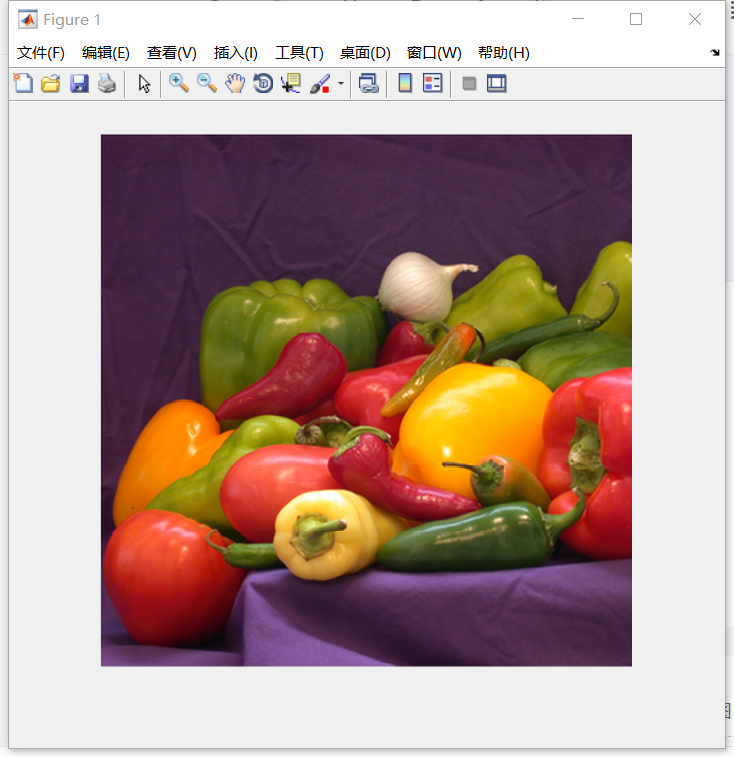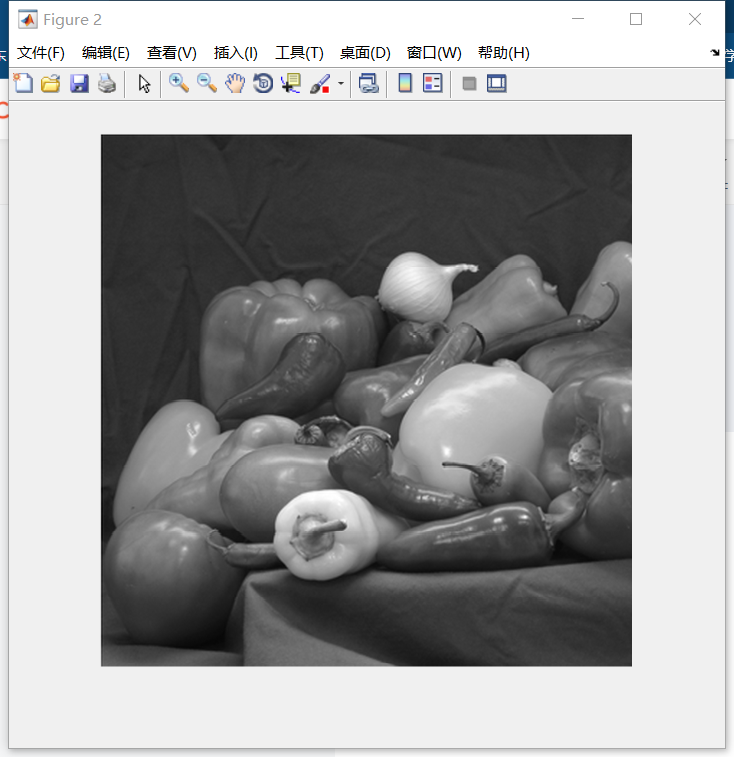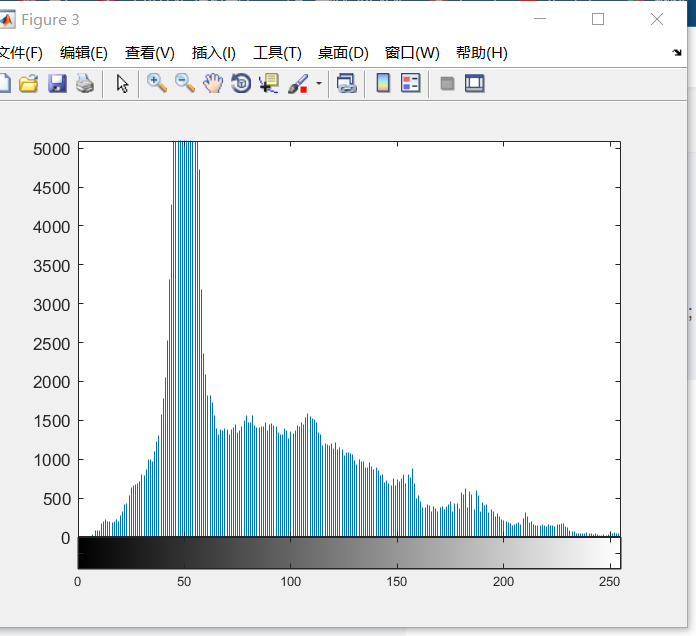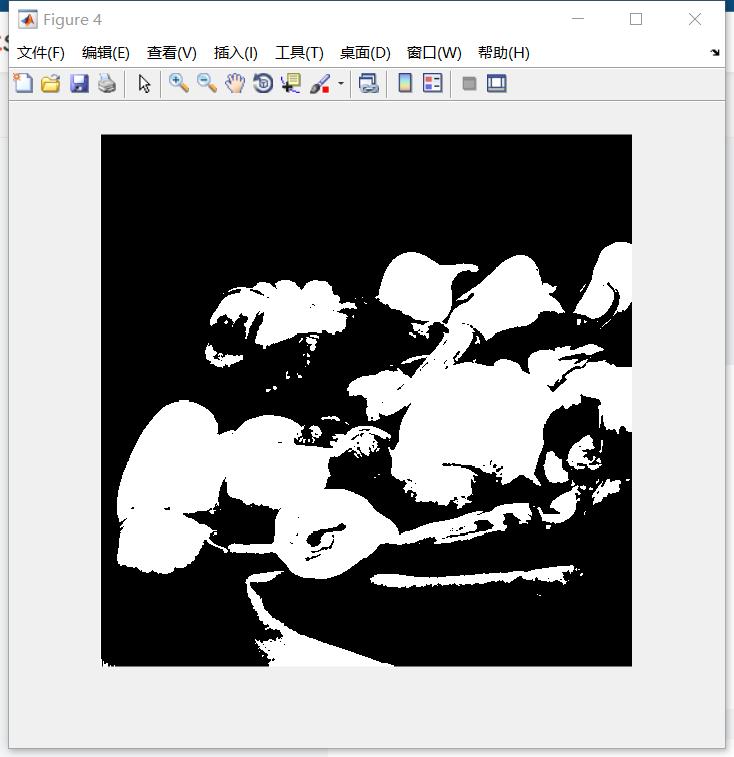2、OTUS阈值分割

T = graythresh(I);  %采用OTSU算法来获取全局阈值，自动选取阈值

K = im2bw(I, T);%二值化

figure;

subplot(121), imshow(I);

subplot(122), imshow(K);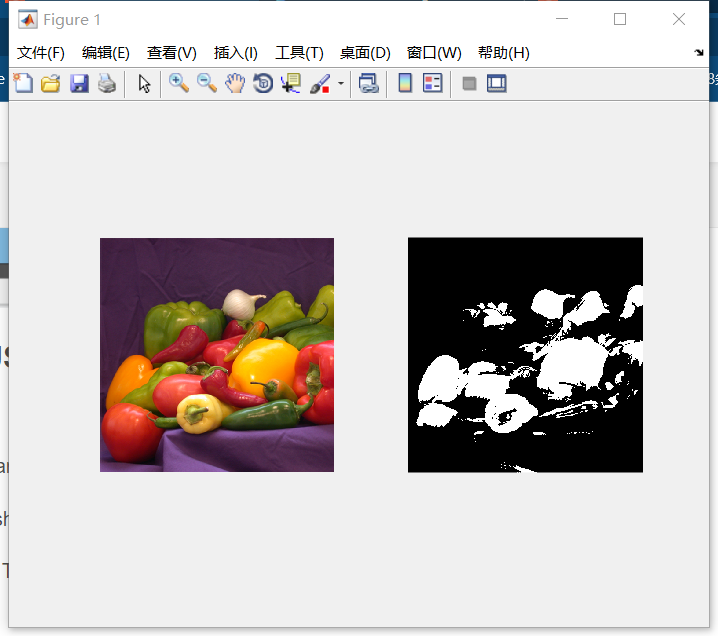展开全文matlab 图像处理 算法 计算机视觉
• 数字图像处理（冈萨雷斯）Matlab版 图像分割部分实例图及相应的处理效果图
• matlab图像分割什么是图像分割固定阈值分割自适应阈值分割分割效果增强平滑处理 什么是图像分割 将数字图像划分成不相交，不重合的区域的过程就叫图像分割。 这很像PhotoShop里面的快速选取工具，判断边界，分割边界...

什么是图像分割

将数字图像划分成不相交，不重合的区域的过程就叫图像分割。
这很像PhotoShop里面的快速选取工具，判断边界，分割边界。

固定阈值分割

先上效果图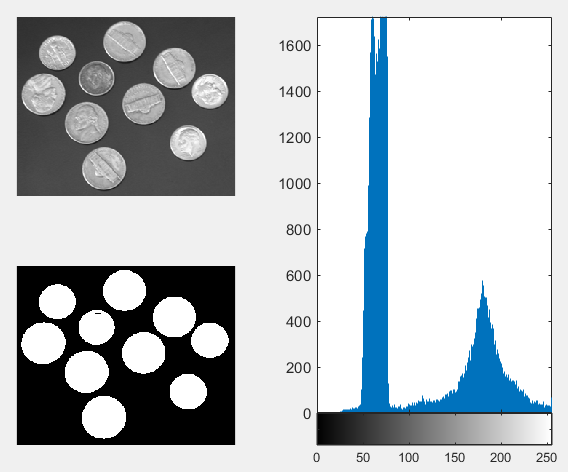这里阈值我手动设置成了0.35，当然，你也可以通过“最大类间方差法”（OTSU）来找到一个最佳阈值。

OTSU：找到灰度直方图中两峰之间的最低点作为阈值。

%固定阈值分割
%原图
subplot(221);imshow(I);

% 直方图
subplot(2,2,[2,4]);imhist(I);

%固定阈值分割，这里阈值为 0.35
level = 0.35;

%im2bw 为分割函数
I_2bw = im2bw(I,level);
subplot(223);imshow(I_2bw);

自适应阈值分割

先上效果图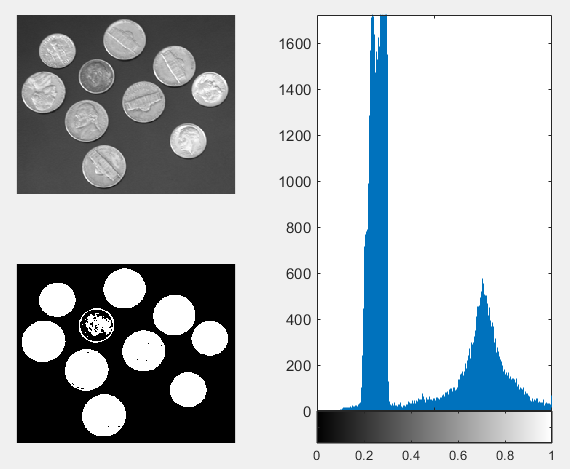采用自适应阈值分割方法比采用直方图分割有更好的分割效果。
对于不同的图像的适应性也更强。

%自适应阈值分割
%原图
subplot(221);imshow(I);
I = im2double(I);

%直方图
subplot(2,2,[2,4]);imhist(I);

diff = 0.01; %设定一个迭代终止值
level1 = ( min(I(:)) + max(I(:)) ) / 2; %初值，当然自己目测一个常数也是可以的
arr1 = find(I>level1);  %第一部分图像
arr2 = find(I<=level1); %第二部分图像
level2 = ( (mean(I(arr1))) + (mean(I(arr2))) ) / 2; %两部分图像取平均
%通过迭代产生最佳阈值
while abs(level1 - level2) < diff
arr1 = find(I>level1);
arr2 = find(I<=level1);
level2 = ( (mean(I(arr1))) + (mean(I(arr2))) ) / 2;
end

%im2bw 为分割函数
I_2bw = im2bw(I,level2);
subplot(223);imshow(I_2bw);

分割效果增强

对于边界不是非常明显的图像，可以对图像进行“平滑处理”后再进行分割。

平滑处理

平滑处理，是增强图像边界的一种方式。

展开全文matlab
• 我数字图像处理的作业，内有实验报告、m文件、图像文件 包括的实验为： 1.Roberts算子、Sobel算子、Prewitt算子、拉普拉斯算子、LoG算子和Canny算子等进行...2.手动阈值分割 3.迭代阈值和OTSU算法对图像进行二值化分割
• 这份代码是实现阈值分割matlab程序，可以直接用。matlab 阈值
• 这两部分都与相连的邻居组件和自适应查找表组合在一起，以实现从背景中实时对移动的手像素进行分割。 依存关系 Kinect2 SDK。 Visual Studio 2012或更高版本的编译器 MATLAB 2013a或更高版本（用于Visual Studio ...
• 它的灵感来自 Matlab 文件交换用户通过 Matlab Answers 解决的多个问题，以及作者的个人页面和电子邮件。 图像处理中分割过程的目标是将图像划分为元素（段）。 当正确定义和实施时，一些元素可能包括场景中有意义...matlab
• 该数据集包含脑部MR图像以及手动FLAIR异常分割蒙版。 这些图像是从The Cancer Imaging Archive（TCIA）获得的。 他们对应于癌症基因组图谱（TCGA）低级神经胶质瘤收集物中的110例患者，至少具有液衰减倒置恢复...
• matlab图像分割（肺实质）

千次阅读 2021-06-16 17:56:51
MATLAB R2010b版本、WIN 7计算机 三、实验内容 读入一幅灰度图像CT.JPG，先分割肺实质区域，然后对感兴趣区域（ 包括肺结节、肺血管和支气管等）进行边缘检测（比较canny算子和marr-Hildreth算子），最后提取感兴趣...

一、实验目的

1. 了解各类边缘的特点；
2. 理解并掌握边缘检测的常用算子。
3. 理解并掌握阈值分割，区域生长，分水岭等分割方法
4. 能够运用以上方法解决实际的图像问题。

二、实验环境

MATLAB R2010b版本、WIN 7计算机

三、实验内容

读入一幅灰度图像CT.JPG，先分割肺实质区域，然后对感兴趣区域（ 包括肺结节、肺血管和支气管等）进行边缘检测（比较canny算子和marr-Hildreth算子），最后提取感兴趣区。给出每步的实验结果图，并对结果进行比较分析。

提示：肺实质的提取可以先对图像进行阈值处理（比较所学的阈值方法，采用最优的），得到二值图像，然后进行形态学操作得到肺实质模板，将模板和CT图像相乘分割得到肺实质图像。感兴趣区域的提取可以运用阈值分割，区域生长，分水岭等方法。

灰度图像CT.JPG阈值分割得到肺实质

分割肺实质 代码

1. 肺实质提取

提取肺实质算法流程：
（1）全局阈值分割
（2）取反
（3）半径为13的圆形结构单元，进行闭运算
（4）取反
（5）孔洞填充
（6）掩膜相减
（7）用掩膜乘原图获得肺实质图像

%%手动阈值分割
clc
clear all;
close all;
subplot(241);imshow(I),title('原始图像');                  %显示图像
J=imhist(I);
subplot(242);imhist(I),title('直方图');            %生成直方图并显示
[M,N]=size(I);                       %返回图像的行数和列数
for i=1:1:M                          %将i以步长11增加到M
for j=1:1:N                      %将j以步长11增加到N
if I(i,j)>80                 %如果图像阈值大于80
g(i,j)=0;                %则大于80的就变成黑的
else g(i,j)=1;               %小于80就变成白的
end
end
end
x=size(g,1);                       %此处分别获得该图片的长度和高度
y=size(g,2);
m=round(y/3);                      %此处进行三等分并取整，因为y/3不一定是整数
for i=1:1:x                        %此处开始三个循环将一个图片分为三个图片
for j=1:1:m
g1(i,j)=g(i,j);
end
end
subplot(243);imshow(g1),title('二值图像');
se2 = strel('disk',17);  % 半径为17的圆形结构元
image2 = imclose(g1,se2);
subplot(2,4,4); imshow(image2); title('经半径为17的圆形结构元闭运算');
tI=~image2;%取反
subplot(2,4,5); imshow(tI); title('取反');
img_fill = imfill(tI, 'holes');
subplot(246),imshow(img_fill), title('孔洞被填充的图像');
img_jian=img_fill-tI;
subplot(247),imshow(img_jian), title('模板');
%% 掩膜*原图
lung1=uint8(Im);
lung2=uint8(img_jian);
lung=immultiply(lung1,lung2);
subplot(248),imshow(lung), title('肺实质');

分割效果边缘检测&感兴趣区域提取

1. 边缘检测

边缘检测（比较canny算子和marr-Hildreth算子）
感兴趣区域的提取可以运用阈值分割，区域生长
代码

%% 边缘检测
figure
I2=edge(lung,'log');
subplot(2,2,1);
imshow(I2);
title('log算子分割结果');
I3=edge(lung,'canny');
subplot(2,2,2);
imshow(I3);
title('canny算子分割结果');Marr-Hildreth算子（LOG算子）没有Canny效果好，不过能够得到闭合的曲线，当然，计算量也比Canny小。LOG算子克服了Laplacian算子抗噪能力差的缺点，但在抑制噪声的同时也将原有的尖锐边缘平滑化了，造成尖锐边缘无法被检测出。Canny算子虽然是基于最优化思想而推导出来的，但是实际效果并不一定最优。同样采用高斯函数对图像平滑化，因此具有较强的抑制噪声能力，但也会将高频边缘平滑掉，造成边缘丢失。Canny算子采用双阈值算法检测和连接边缘，采用的多尺度检测和方向性搜索比LOG算子好。

1. 阈值分割

通过下列代码选择特定区域观察其直方图，确定人工选定的阈值

%% 阈值分割提取感兴趣区域
[M,N]=size(lung);                       %返回图像的行数和列数
subplot(223),imshow(lung), title('肺实质');
rect=getrect();
B=imcrop(lung,rect);
subplot(224);imhist(B),title('直方图');

%% 人工选定阈值进行分割，选择阈值为68
figure
T1=68;
for i=1:1:M                          %将i以步长11增加到M
for j=1:1:N                      %将j以步长11增加到N
if lung(i,j)>T1                 %如果图像阈值大于68
lg(i,j)=0;                %则大于68的就变成黑的
else lg(i,j)=1;               %小于68就变成白的
end
end
end
subplot(2,2,1);imshow(lg),title('肺血管');                  %保持原图，显示图像g

阈值处理提取感兴趣的区域（手动阈值分割的阈值是取直方图中双峰的谷底的灰度值作为阈值，若有多个双峰谷底，则取第一个作为阈值），经过阈值分割后肺部血管和肺实质其他部分可以分隔开，但由于此法误差较大所以用来观察血管内是个否存在栓塞不够准确。

1. 区域生长
通过下列代码作区域生长，提取感兴趣区域
%% 区域生长

J=regionGrow(lung);
imshow(J),title('区域生长');

%% 区域生长函数
function J=regionGrow(I)
if isinteger(I)
I=im2double(I);
end
figure
imshow(I)
[M,N]=size(I);
[y,x]=getpts;%单击取点后，按enter结束
x1=round(x);
y1=round(y);
seed=I(x1,y1);
J=zeros(M,N);
J(x1,y1)=1;
sum=seed;
suit=1;%点的个数
count=1;
threshold=0.15;
while count>0
s=0;
count=0;
for i=1:M
for j=1:N
if J(i,j)==1
if (i-1)>0&(i+1)<(M+1)&(j-1)>0&(j+1)<(N+1)
for u=-1:1
for v=-1:1
if J(i+u,j+v)==0&abs(I(i+u,j+v)-seed)<=threshold&1/(1+1/15*abs(I(i+u,j+v)-seed))>0.8
J(i+u,j+v)=1;
count=count+1;
s=s+I(i+u,j+v);
end
end
end
end
end
end
end
suit=suit+count;
sum=sum+s;
seed=sum/suit;
end
end

分割效果考虑区域生长算法，将相似的像素点聚合成更大区域。分割图像时可以用鼠标在其中选取一个种子点并按下回车键，之后会出现分割结果。应用区域生长算法，可以对某段血管内是否有栓塞进行精细判断，但由于种子点选取原因，效率较慢。另外，区域生长对于该区域与外界区域有明显差距的图像，分割效果很好，而对感兴趣区域与外接区域存在边缘连通现象的图像，分割效果很差，如分割不完整、过分割等。

展开全文matlab 图像处理
• matlab图像分割肿瘤代码小鼠胶质母细胞瘤肿瘤深度分割的深度学习 __0.94 DSC（准确度为94％）____________________预计的细分_____手动细分 。 。 。 方法 小鼠胶质母细胞瘤模型 这项研究的所有程序和影像学方案均已...
• AudioAnnotation Demo v.1.0 是在 Matlab(R) 中实现的开源演示，用于手动分割和注释音频文件。 您可以将其用于定义基本事实，以检查细分分类算法的性能。 它还提供了计算和绘制所选音频片段的基本音频特征（例如，...matlab
• -覆盖多标签遮罩-手动ROI工具-测量工具（面积，距离，强度分布图） -有效轮廓，切片插值以实现ROI -灰度或RGB颜色，伽玛校正-直方图，统计-鼠标控制 * imtool3D：标准查看器* imtool3D_3planes：3个切片平面查看器* ...matlab
• title('3lena由matlab直方图'); %直方图自己算 [width,height,bmsize]=size(wangpicgray); myzjhist=zeros(256,1); myquzhi=0; for i=1:width for j=1:height myquzhi=wangpicgray(i,j); myzjhist(myquzhi+1,1)=...

close all;%关闭所有窗口

clear;%清除变量的状态数据

clc;%清除命令行

figure;%打开一个新窗口

imshow(wangpic);

title('1 lena的原图');

wangpicgray=rgb2gray(wangpic);

figure;%打开一个新窗口

imshow(wangpicgray);

title('2lena的灰度图');

%直方图

figure;%打开一个新窗口

imhist(wangpicgray);

title('3lena由matlab直方图');

%直方图自己算

[width,height,bmsize]=size(wangpicgray);

myzjhist=zeros(256,1);

myquzhi=0;

for i=1:width

for j=1:height

myquzhi=wangpicgray(i,j);

myzjhist(myquzhi+1,1)=myzjhist(myquzhi+1,1)+1;

end

end

figure;

plot(myzjhist);

title('4 lena自己计算直方图');

%阈值

[width,height,bmsize]=size(wangpicgray);

for i=1:width

for j=1:height

if wangpicgray(i,j)>140

wangpicgray(i,j)=255;

else

wangpicgray(i,j)=0;

end

end

end

figure;

imshow(wangpicgray);

title('5lena二值化');

figure;%打开一个新窗口

imshow(wanpic);

title('6瓜子的原图');

wanpicgray=rgb2gray(wanpic);

figure;%打开一个新窗口

imshow(wanpicgray);

title('7瓜子的灰度图');

%直方图

figure;%打开一个新窗口

imhist(wanpicgray);

title('8 瓜子由matlab直方图');

%直方图自己算

[width,height,bmsize]=size(wanpicgray);

myzjhistguazi=zeros(256,1);

myquzhiguazi=0;

for i=1:width

for j=1:height

myquzhiguazi=wanpicgray(i,j);

myzjhistguazi(myquzhiguazi+1,1)=myzjhistguazi(myquzhiguazi+1,1)+1;

end

end

figure;

plot(myzjhistguazi);

title('9 瓜子自己计算直方图');

%阈值 [width,height,bmsize]=size(wanpicgray); for i=1:width     for j=1:height         if wanpicgray(i,j)>140             wanpicgray(i,j)=255;         else             wanpicgray(i,j)=0;         end     end end   figure; imshow(wanpicgray); title('10 瓜子二值化');

展开全文• %手动输入阈值 % p3 = inputdlg(p1,'Input Num:1~256',1,p2); % p = str2num(p3{1}); p = p/255; %% 图片分割 image = im2bw(I, p); %小于阈值的为黑，大于阈值的为白 subplot(2, 2, 2); imshow(image); title('（b...
• 眼睛血管分割matlab版本

千次阅读 热门讨论 2019-10-27 20:37:14
matlab的代码仅供参考，实现过程基本按照python版本的眼睛血管分割来实现。其中，python版本眼睛血管分割详见：https://blog.csdn.net/qq_40511157/article/details/102770108 clc; clear all; close all; image=...matlab
• 颅骨分割matlab代码SLS（Salem病变分割） 关于： SLS工具箱的实现最初是用C ++开发到Matlab中的。 性能测试： 我们已经针对原始C ++代码评估了Matlab实现的性能。 比较测试是使用Vall d'Hebron医院中心提供的70幅...
• 基于MATLAB的图像分割系统GUI界面一、课题介绍　图像分割作为图像分析和理解的一个关键步骤，其结果将直接影响到目标物特征提取和描述，以及进一步的目标物识别、分类和图像理解。本文全面细致地介绍了图像的分割...算法 python 计算机视觉 机器学习 人工智能
• 实现多光谱图像的分割手动采点MATLAB，对任意点位置均可，亲测绝对可用，读入的图片要换分别为多光谱图和PS处理后的多光谱图。采样量化
• 区域生长算法原理及MATLAB实现 【C++】pcl中的Region Growing（区域生长）算法 区域生长的原理 1、排序，基于曲率对点云进行排序，曲率最小的点叫做初始种子点，区域生长算法从曲率最小的种子点开始生长，初始...区域生长 点云分割 matlab
• matlab图像分割肿瘤代码斯坦福大学-机器学习 在本课程中，我们涵盖以下主题： 第一周 介绍 一变量线性回归 线性代数复习 第二周 多元线性回归 八度/ Matlab教程 第三周 逻辑回归 正则化 第四周神经网络：表示 第五周...
• 手动进行人脸特征点标定的matlab程序，使用起来非常的方便，且可自动生成特征点存储文件。
• MATLAB图像处理之人工阈值分割 %人工阈值分割 football = imread('football.jpg'); a=rgb2gray('football'); imhist(a); %灰度直方图 [m,n]=size(a); football=a; for i=1:m for j=1:n ...matlab
• matlab提取轮廓代码脸部视频分割-视频中的脸部分割地面真相 面部视频分段编辑器的快照。 ， ， ， ， 和 。 概述 该项目包含用于从视频中半监督收集地面真实面Kong分割数据的工具集合。 使用的方法从密集的光流中......

matlab手动分割matlab 订阅